# Class 12 Maths NCERT Solutions for Chapter 11 Three Dimensional Geometry Exercise 11.1### Three Dimensional Geometry Exercise 11.1 Solutions

1. If a line makes angles 90°, 135°, 45° with the x, y and z axes respectively, find its direction cosines.

Solution

Let direction cosines of the line be l, m, and n.
I = cos 90° = 0
m = cos 135° = -1/√2
n = cos 45° = 1/√2
Therefore, the direction cosines of the line are 0, -1/√2  and 1/√2.

2. Find the direction cosines of a line which makes equal angles with the coordinate axes.

Solution

Let the direction cosines of the line make an angle a with each of the coordinate axes.
∴ I = cos α, m = cos α, n = cos α
l2 + m2 + n2 = 1
⇒ cos2 α + cosα + cos2 α = 1
⇒ 3 cos2 α = 1
⇒ cos2 α = 1/3
⇒ cos α = ± 1/√3
Thus, the direction cosines of the line, which is equally inclined to the coordinate axes, are ± 1/√3, ± 1/√3, and ± 1/√3.

3. If a line has the direction ratios −18, 12, −4, then what are its direction cosines?

Solution

If a line has direction ratios of -18, 12 and -4, then its direction cosines are: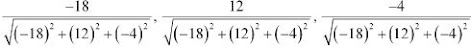i.e., -18/22 , 12/22, -4/22
-9/11, 6/11, -2/11
Thus, the direction cosines are -9/11, 6/11, and -2/11.

4. Show that the points (2, 3, 4), (-1, -2, 1), (5, 8, 7) are collinear.

Solution

The given points are A (2, 3, 4), B (−1, −2, 1), and C (5, 8, 7).
It is known that the direction ratios of line joining the points, (x1y1z1) and (x2y2z2), are given by, x2 − x1y2 − y1, and z2 − z1.
The direction ratios of AB are (-1 −2), (−2 −3), and (1 −4) i.e., −3, −5, and −3.
The direction ratios of BC are (5 −(−1)), (8 −(−2)), and (7 − 1) i.e., 6, 10, and 6.
It can be seen that the direction ratios of BC are −2 times that of AB i.e., they are proportional.
Therefore, AB is parallel to BC. Since point B is common to both AB and BC, points A, B, and C are collinear.

5. Find the direction cosines of the sides of the triangle whose vertices are (3, 5, −4), (−1, 1, 2) and (−5, −5, −2)

Solution

The vertices of Δ ABC are A(3, 5, -4), B(-1, 1, 2) and C(-5, -5, -2).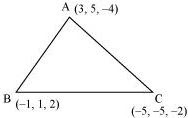The direction ratios of side AB are (−1 − 3), (1 − 5), and (2 − (−4)) i.e., −4, −4, and 6.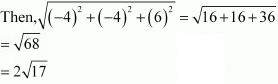Therefore, the direction cosines of AB are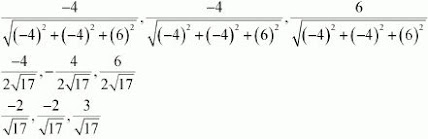The direction ratios of BC are (−5 −(−1)), (−5 −1), and (−2 −2) i.e., −4, −6, and −4.
Therefore, the direction cosines of BC are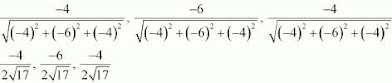-217, -317, -217The direction ratios of CA are 3−(−5), 5−(−5) and −4−(−2) i.e. 8, 10 and -2.
Therefore the direction cosines of CA are 882 + 102 +(-22), 1082 + 102 + -22, -282 + 102 + (-228242), 10242, -2242442, 542, -142